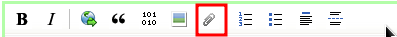# Add labels to data in histogram (Calc)

Greetings,

I have a Calc sheet with data in columns. I have created an histogram with data in one column (say D) as horizontal axis and data in another column (say G) and vertical axis. Now, I would like to add labels to the histogram. How can I do that?
Let me explain with an example.

Column D contains x-values

Column G contains y-values

The histogram shows y = f(x)

Next, I have labels for y in column - say - R. I would like to include column R in the histogram so that above each bar of the histogram the corresponding value in column R is shown.

Any idea?

In fact I don’t understand your question. A histogram (column type of chart) doesn’t show a functional dependency but categories (which is labels in your terms) on the x-axis. Hence either your column `D` constitutes the x-axis or column `R`. Your question seems like: I’d like to have a second x-axis for visual mapping of column `D` categories to column `R` categories. Could you provide a sample file?